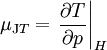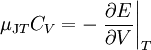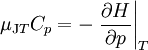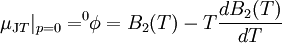# Joule-Thomson effect

The Joule-Thomson effect is also known as the Joule-Kelvin effect. This effect is present in non ideal gasses, where a change in temperature occurs upon expansion.

## Joule-Thomson coefficient

The Joule-Thomson coefficient is given by$\mu_{\mathrm JT} = \left. \frac{\partial T}{\partial p} \right\vert_H$

where T is the temperature, p is the pressure and H is the enthalpy.

In terms of heat capacities one has$\mu_{\mathrm JT} C_V = -\left. \frac{\partial E}{\partial V} \right\vert_T$

and$\mu_{\mathrm JT} C_p = -\left. \frac{\partial H}{\partial p} \right\vert_T$

In terms of the second virial coefficient at zero pressure one has$\mu_{\mathrm JT}\vert_{p=0} = ^0\!\!\phi = B_2(T) -T \frac{dB_2(T)}{dT}$